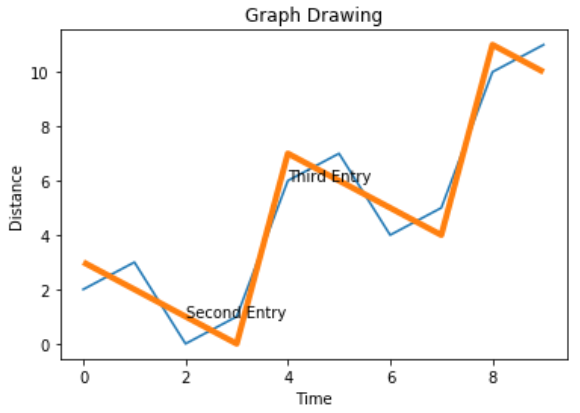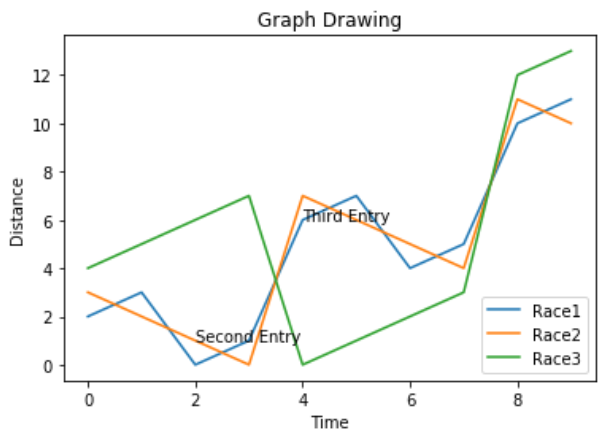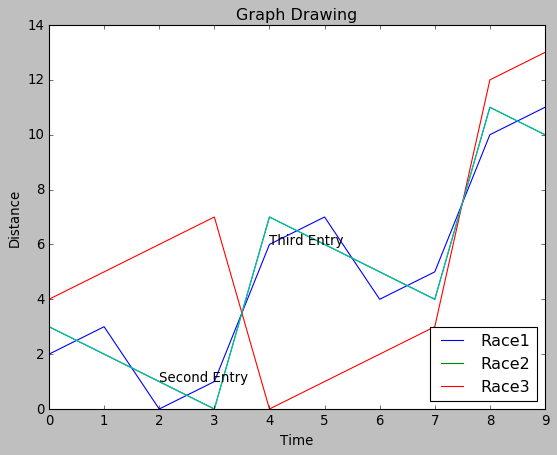# Python - Chart Styling

#### Beyond Basic Programming - Intermediate Python

Most Popular

36 Lectures 3 hours

#### Practical Machine Learning using Python

Best Seller

91 Lectures 23.5 hours

#### Practical Data Science using Python

22 Lectures 6 hours

The charts created in python can have further styling by using some appropriate methods from the libraries used for charting. In this lesson we will see the implementation of Annotation, legends and chart background. We will continue to use the code from the last chapter and modify it to add these styles to the chart.

Many times, we need to annotate the chart by highlighting the specific locations of the chart. In the below example we indicate the sharp change in values in the chart by adding annotations at those points.

```import numpy as np
from matplotlib import pyplot as plt

x = np.arange(0,10)
y = x ^ 2
z = x ^ 3
t = x ^ 4
# Labeling the Axes and Title
plt.title("Graph Drawing")
plt.xlabel("Time")
plt.ylabel("Distance")
plt.plot(x,y)

#Annotate
plt.annotate(xy=[2,1], s='Second Entry')
plt.annotate(xy=[4,6], s='Third Entry')
```

Its output is as follows −We sometimes need a chart with multiple lines being plotted. Use of legend represents the meaning associated with each line. In the below chart we have 3 lines with appropriate legends.

```import numpy as np
from matplotlib import pyplot as plt

x = np.arange(0,10)
y = x ^ 2
z = x ^ 3
t = x ^ 4
# Labeling the Axes and Title
plt.title("Graph Drawing")
plt.xlabel("Time")
plt.ylabel("Distance")
plt.plot(x,y)

#Annotate
plt.annotate(xy=[2,1], s='Second Entry')
plt.annotate(xy=[4,6], s='Third Entry')
plt.plot(x,z)
plt.plot(x,t)
plt.legend(['Race1', 'Race2','Race3'], loc=4)
```

Its output is as follows −## Chart presentation Style

We can modify the presentation style of the chart by using different methods from the style package.

```import numpy as np
from matplotlib import pyplot as plt

x = np.arange(0,10)
y = x ^ 2
z = x ^ 3
t = x ^ 4
# Labeling the Axes and Title
plt.title("Graph Drawing")
plt.xlabel("Time")
plt.ylabel("Distance")
plt.plot(x,y)

#Annotate
plt.annotate(xy=[2,1], s='Second Entry')
plt.annotate(xy=[4,6], s='Third Entry')